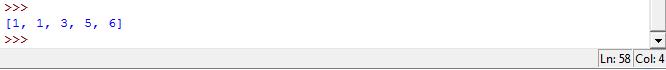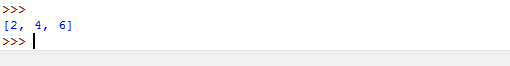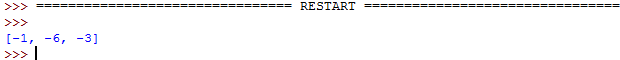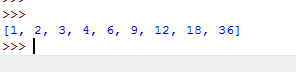# Answered! Python 3 help!!! 1.Given the lists, lst1 and lst2, create a new sorted list consisting of all the elements of lst1 that do not appear in lst2…

Python 3 help!!!

1.Given the lists, lst1 and lst2, create a new sorted list consisting of all the elements of lst1 that do not appear in lst2 together with all the elements of lst2 that do not appear in lst1. For example, if lst1 is [4, 3, 2, 6, 2] and lst2 is [1, 2, 4, 1, 5], then the new list would be [1, 1, 3, 5, 6]. Note that duplicate elements are also duplicated in the new list. Associate the new list with the variable new_list, and don’t forget to sort the new list.

Don't use plagiarized sources. Get Your Custom Essay on
Answered! Python 3 help!!! 1.Given the lists, lst1 and lst2, create a new sorted list consisting of all the elements of lst1 that do not appear in lst2…
GET AN ESSAY WRITTEN FOR YOU FROM AS LOW AS \$13/PAGE

2.Given the list my_list containing integers, create a list consisting of all the even elements of my_list. Associate the new list with the variable new_list.

3.Create a list consisting of the negative values in the list numbers. Associate the new list with the variable negatives.

4.The factors of an integer are those numbers that evenly divide into the integer. Given the integer n, create a list of all the factors of n, excluding 1 and nitself. The list should be ordered from smallest to largest. Associate the new list with the variable factors.

1. Check using for loop if a given element in one list is present in another. Finalls take union of both lists.

Code:

list1=[4,3,2,6,2]
list2=[1,2,4,1,5]
list1dif=[]
list2dif=[]

#Check if element in list1 is not present in list2
for i in list1:
flag=0
for j in list2:
if i==j:
flag=1
#if element not present, append it.
if flag!=1:
list1dif.append(i)

#Check if element in list2 is not present in list1
for i in list2:
flag=0
for j in list1:
if i==j:
flag=1
#if element not present, append it.
if flag!=1:
list2dif.append(i)

#finally sort the union of both lists
new_list=sorted(list1dif+list2dif)

print new_list

Output :2. If number in list is divisble by 2, append it in new_list

Code:

my_list=[1,2,3,4,5,6]
new_list=[]
for i in my_list:
if i%2==0:
new_list.append(i)
print new_list

Output :3. If a number is less than 0 in the list, append it in list negatives.

Code :

l=[-1,2,4,-6,-3,7]
negatives=[]
for i in l:
if i<0:
negatives.append(i)
print negatives

Output:4. Check if number is divisible upto the range n/2 (including the number n/2). Also append the number iteself in the list factors.

Code :

n=36
factors=[]
for i in range(1,n/2+1): #n/2 + 1 signifies the integer n/2 included. In this case 18.
if n%i==0:
factors.append(i)
factors.append(n) #append the number itself
print factors

Output: